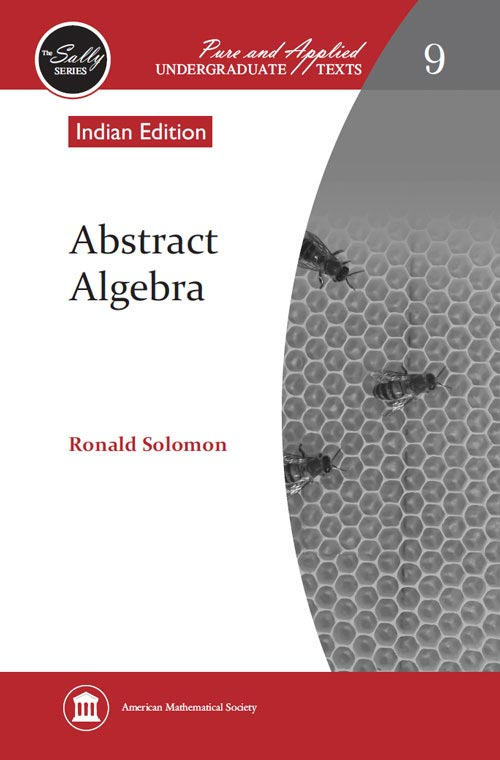Abstract Algebra
Ronald Solomon
Price
1075.00
ISBN
9780821852101
Language
English
Pages
240
Format
Paperback
Dimensions
158 x 240 mm
Year of Publishing
2010
Territorial Rights
Restricted
Imprint
American Mathematical Society
Catalogues

At the heart of the text is a semi-historical journey through the early decades of the subject as it emerged in the revolutionary work of Euler, Lagrange, Gauss, and Galois. Avoiding excessive abstraction whenever possible, the text focuses on the central problem of studying the solutions of polynomial equations. Highlights include a proof of the Fundamental Theorem of Algebra, essentially due to Euler, and a proof of the constructability of the regular 17-gon, in the manner of Gauss. Another novel feature is the introduction of groups through a meditation on the meaning of congruence in the work of Euclid. Everywhere in the text, the goal is to make clear the links connecting abstract algebra to Euclidean geometry, high school algebra, and trigonometry. Another goal is to encourage students, insofar as possible in a textbook format, to build the course for themselves, with exercises integrally embedded in the text of each chapter.

Ronald Solomon, Ohio State University, Columbus, Ohio, USA

Section 1: Geometry

• What Is Congruence?
• Some Two-Dimensional Geometry
• Symmetry

Section 2: Polynomials

• The Root of It All
• The Renaissance of Algebra
• Complex Numbers
• Symmetric Polynomials and The Fundamental Theorem of Algebra
• Permutations and Lagrange’s Theorem
• Orbits and Cauchy’s Formula
• Hamilton’s Quaternions (Optional)

Section 3: Numbers

• Back to Euclid; Euclid’s Lemma for Polynomials; Fermat and the Rebirth of Number Theory
• Lagrange’s Theorem Revisited; Rings and Squares
• More Rings and More Squares
• Fermat’s Last Theorem (for Polynomials)
• Still more Fermat’s Last Theorem (Optional)

Section 4: The Grand Synthesis

• Constructible Polygons and the Method of Mr. Gauss
• Cyclotomic Fields and Linear Algebra
• Lagrange Theorem for Fields and Nonconstructibility
• Galois Fields and the Fundamental Theorem of Algebra Revisited
• Galois’ Theory of Equations
• The Galois Correspondence
• Constructible Numbers and Solvable Equations
THE BOOKPOINT (INDIA) PVT. LTD.